Exam Mission

Exam Information| Govt Jobs.| Exam Preparation | Exam Study Material |Exam Date|Material PDF

1. Trigonometry:
In a right angled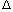OAB, where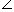BOA =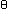,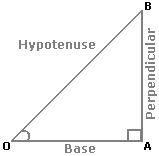i.   sin= Perpendicular = AB ; Hypotenuse OB
 ii.   cos= Base = OA ; Hypotenuse OB
 iii.  tan= Perpendicular = AB ; Base OA
 iv.  cosec= 1 = OB ; sinAB
 v.   sec= 1 = OB ; cosOA
 vi.  cot= 1 = OA ; tanAB
2. Trigonometrical Identities:
1. sin2+ cos2= 1.
2. 1 + tan2= sec2.
3. 1 + cot2= cosec2.
3. Values of T-ratios:(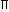/6)

30°
(/4)

45°
(/3)

60°
(/2)

90°
sin0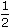1 2
 3 2
1
cos1
 3 2
 1 20
tan0
 1 3
13not defined
4. Angle of Elevation: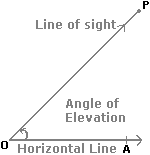Suppose a man from a point O looks up at an object P, placed above the level of his eye. Then, the angle which the line of sight makes with the horizontal through O, is called the angle of elevation of P as seen from O.Angle of elevation of P from O =AOP.
5. Angle of Depression: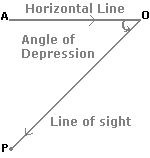Suppose a man from a point O looks down at an object P, placed below the level of his eye, then the angle which the line of sight makes with the horizontal through O, is called the angle of depression of P as seen from O.